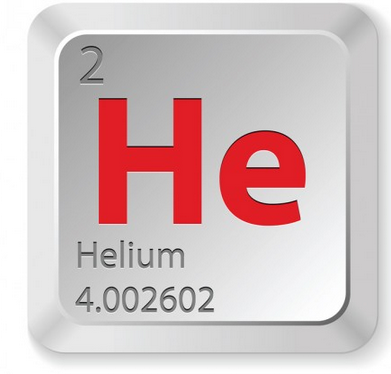# Calculate the mass of 1.23 * 10^24 helium atoms?

1.23 X 10^24 helium atoms (1 mole He atoms/6.022 X 10^23)(4.003 grams/1 mole He)

= 8.18 grams helium

======

thanked the writer.

The calculation needed to work out the mass of 1.23*10^24 of Helium atoms is a lot simpler if you leave out the 10^24 until the end.According to the Harvard table of physical and astronomical constants, the weight of 1 atom of helium is 4.00260325415 amu, where 1 amu is 1.6605402-10^24 grams. Therefore, we can find out the weight of 1 helium atom in grams as follows:

• 4.00260325415 x 1.6605402-10^24 grams = 6.64648360817-10^24 grams.
• Your question requires us to calculate the mass of 1.23*10^24 helium atoms. 10^24 written out is 1,000,000,000,000,000,000,000,000 and so:
• 1.23 x 1,000,000,000,000,000,000,000,000 = 1,230,000,000,000,000,000,000,000 atoms.
• Or, you could write out the number as 1.23 septillion.
• However, as the weight of 1 helium atom is measured in -10^24, and we are being asked to find the weight of 1.23*10^24, then one cancels out the other, leaving us a straightforward sum:
• 1.23 x 6.64648360817 = 8.1751748305.
• Therefore, the weight of 1.23 septillion helium atoms equals 8.1751748305 grams, or to round up, 8.18 grams.

For similar weighty questions on Blurtit, take a look at Calculate The Mass In Grams Of A Single Water Molecule? or Calculate The Formula Of Atoms In 0.377 Grams Of Ar?

YouTube is a good place to take a few science lessons, and can find out more about the mass of helium by watching this film:

thanked the writer.